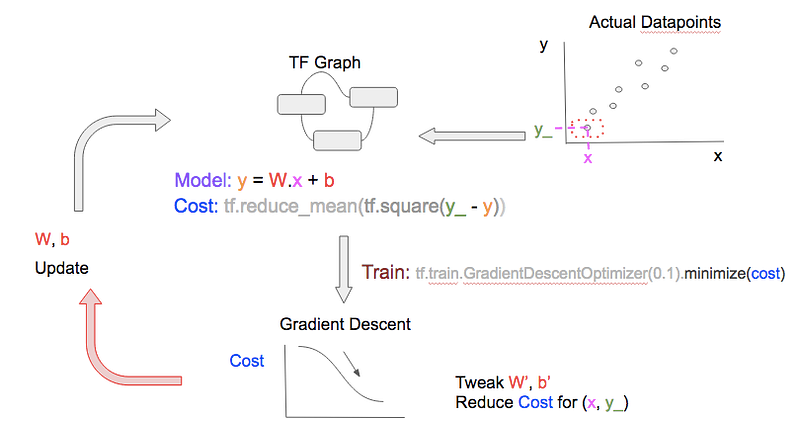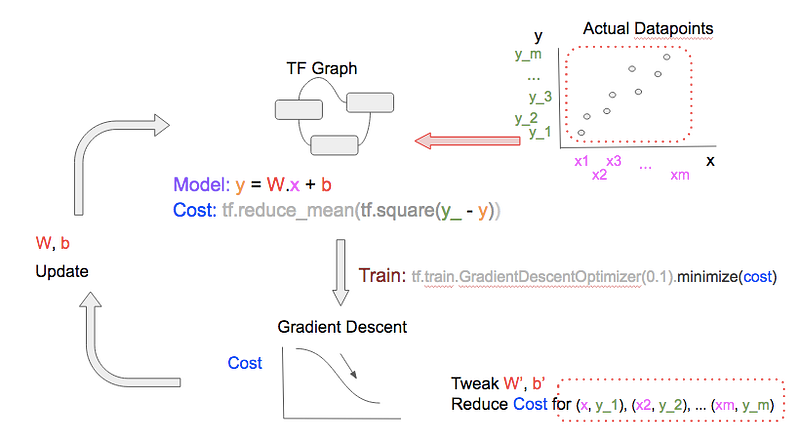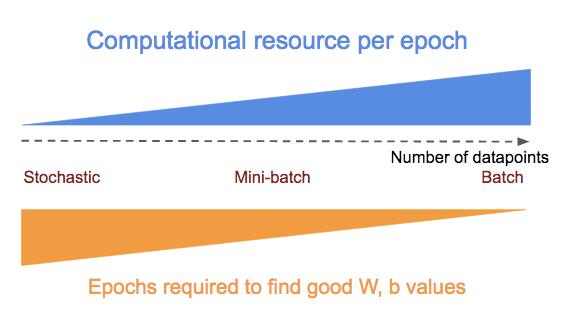Submit a blog to KDnuggets -- Top Blogs Win A Reward

Topics: AI | Data Science | Data Visualization | Deep Learning | Machine Learning | NLP | Python | R | Statistics

KDnuggets Home » News » 2016 » Aug » Tutorials, Overviews » The Gentlest Introduction to Tensorflow – Part 2 ( 16:n31 )

# The Gentlest Introduction to Tensorflow – Part 2

Check out the second and final part of this introductory tutorial to TensorFlow.

### Training Variation

Stochastic, Mini-batch, Batch

In the training above, we feed a single datapoint at each epoch. This is known as stochastic gradient descent. We can feed a bunch of datapoints at each epoch, which is known as mini-batch gradient descent, or even feed all the datapoints at each epoch, known as batch gradient descent. See the graphical comparison below and note the 2 differences between the 3 diagrams:

• The number of datapoints (upper-right of each diagram) fed to TF.Graph at each epoch
• The number of datapoints for the gradient descent optimizer to consider when tweaking W, b to reduce cost (bottom-right of each diagram)The number of datapoints used at each epoch has 2 implications. With more datapoints:

• Computational resource (subtractions, squares, and additions) needed to calculate the cost and perform gradient descent increases
• Speed at which the model can learn and generalize increases

The pros and cons of doing stochastic, mini-batch, batch gradient descent can be summarized in the diagram below:Pros and cons of stochastic, mini-batch & batch gradient descent

To switch between stochastic/mini-batch/batch gradient descent, we just need to prepare the datapoints into different batch sizes before feeding them into the training step [D], i.e., use the snippet below for[C]:

```# * all_xs: All the feature values
# * all_ys: All the outcome values
# datapoint_size: Number of points/entries in all_xs/all_ys
# batch_size: Configure this to:
#             1: stochastic mode
#             integer < datapoint_size: mini-batch mode
#             datapoint_size: batch mode
# i: Current epoch number

if datapoint_size == batch_size:
# Batch mode so select all points starting from index 0
batch_start_idx = 0
elif datapoint_size < batch_size:
# Not possible
raise ValueError(“datapoint_size: %d, must be greater than
batch_size: %d” % (datapoint_size, batch_size))
else:
# stochastic/mini-batch mode: Select datapoints in batches
#                             from all possible datapoints
batch_start_idx = (i * batch_size) % (datapoint_size — batch_size)
batch_end_idx = batch_start_idx + batch_size
batch_xs = all_xs[batch_start_idx:batch_end_idx]
batch_ys = all_ys[batch_start_idx:batch_end_idx]

# Get batched datapoints into xs, ys, which is fed into
# 'train_step'
xs = np.array(batch_xs)
ys = np.array(batch_ys)```

Learn Rate Variation

Learn rate is how big an increment/decrement we want gradient descent to tweak W, b, once it decides whether to increment/decrement them. With a small learn rate, we will proceed slowly but surely towards minimal cost, but with a larger learn rate, we can reach the minimal cost faster, but at the risk of ‘overshooting’, and never finding it.

To overcome this, many ML practitioners use a large learn rate initially (with the assumption that initial cost is far away from minimum), and then decrease the learn rate gradually after each epoch.

TF provides 2 ways to do so as wonderfully explained in this StackOverflow thread, but here is the summary.

Use tf.placeholder for Learn Rate

As you have learned previously, if we declare a tf.placeholder, in this case for learn rate, and use it within the tf.train.GradientDescentOptimizer, we can feed a different value to it at each training epoch, much like how we feed different datapoints to x, y_, which are also tf.placeholders, at each epoch.

We need 2 slight modifications:

```# Modify [B] to make 'learn_rate' a 'tf.placeholder'
# and supply it to the 'learning_rate' parameter name of
learn_rate = tf.placeholder(tf.float32, shape=[])
learning_rate=learn_rate).minimize(cost)

# Modify [D] to include feed a 'learn_rate' value,
# which is the 'initial_learn_rate' divided by
# 'i' (current epoch number)
# NOTE: Oversimplified. For example only.
feed = { x: xs, y_: ys, learn_rate: initial_learn_rate/i }
sess.run(train_step, feed_dict=feed)```

### Wrapping Up

We illustrated what machine learning ‘training’ is, and how to perform it using Tensorflow with just model & cost definitions, and looping through the training step, which feeds datapoints into the gradient descent optimizer. We also discussed the common variations in training, namely changing the size of datapoints the model uses for learning at each epoch, and varying the learn rate of gradient descent optimizer.

Coming Up Next

• Set up Tensor Board to visualize TF execution to detect problems in our model, cost function, or gradient descent
• Perform linear regression with multiple features

Resources

Bio: Soon Hin Khor, Ph.D is using tech to make the world more caring, and responsible. Contributor of ruby-tensorflow. Co-organizer for Tokyo Tensorflow meetup.

Original. Reposted with permission.

Related:

## Top Stories Past 30 Days

Most Popular
Most SharedGet KDnuggets, a leading newsletter on AI, Data Science, and Machine Learning